With new enhanced and efficient editing capabilities, you can now make the most of your spreadsheet in Google Docs. Now writing formulas and applying functions are as easy as in Excel. Google Docs recently added new functionality that will allow you to formulate datasets instantly. In this post we will be putting some light on using formulas and functions in Google Docs Datasheet.

Along with validating data, inserting gadgets and adding  new sheets,  you will see another addition – Formula Bar.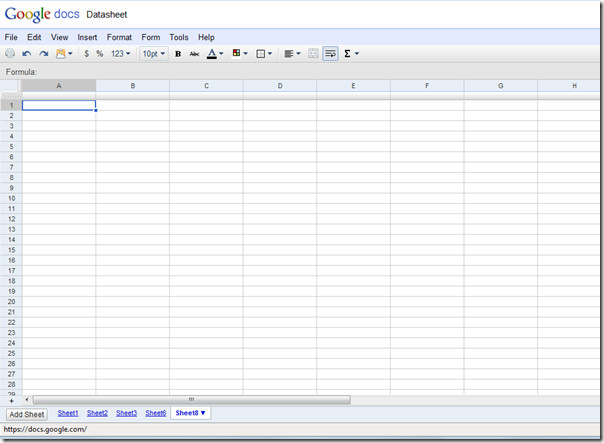By default, the formula bar is hidden, to make it apparent go to View menu and click Show formula bar.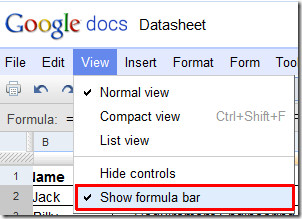Now lets try to make an easy Google Docs Spreadsheet containing formulas and functions. We will be creating a student grading system in it, having fields Name, Course, Marks, Grade and Prize Money.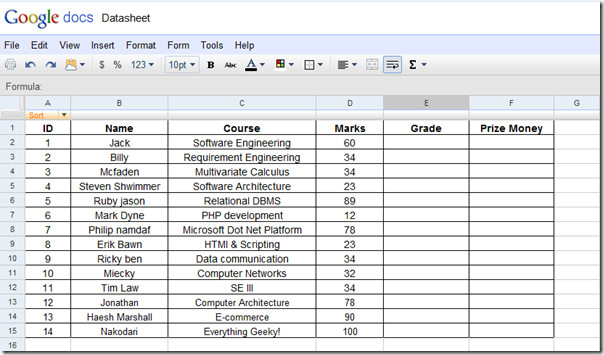Our main emphasize will be on Grade and Prize Money field, where we will be evaluating the grades secured by students and their resultant prize money. We have also included a criteria field in the same spreadsheet.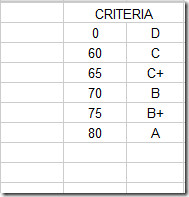For evaluating grades we will be writing VLOOKUP function in first column as;

=VLOOKUP(D2,\$H\$1:\$I\$6,2,TRUE)

While applying formula in the spreadsheet, it will show all recommendations by displaying the complete syntax of it. It will automatically yield the desired result, as shown in the screenshot below.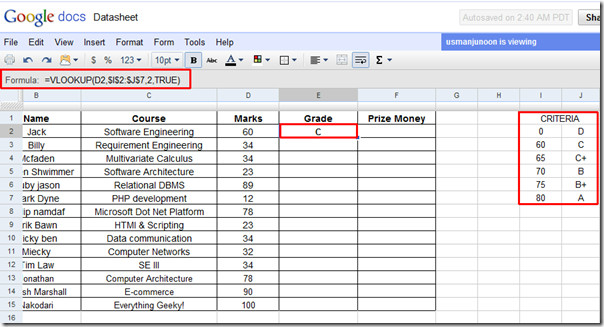Now drag the plus sign towards the end of the column to apply it over.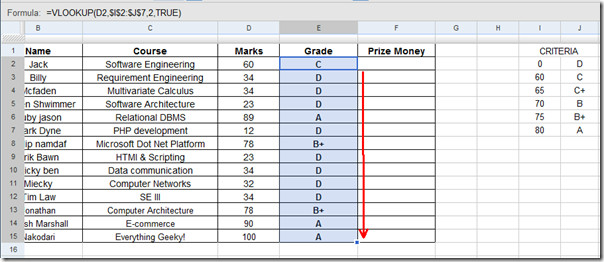For evaluating prize money, we have also included a criteria table. Just repeat the procedure of applying VLOOKUP formula as we did in the Grade column.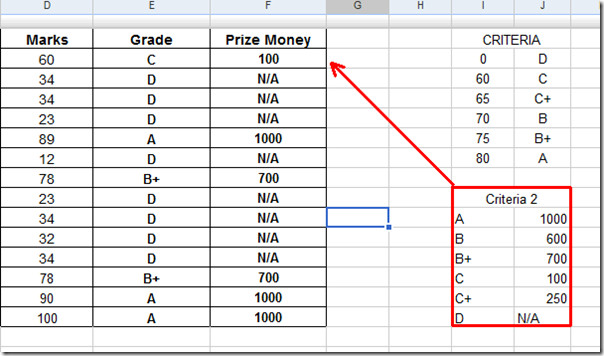Now we will evaluate Status of the students that will either be Pass or Fail. For this we will be adding a simple formula, which will check Grade field values against it and show the status.

=IF(E2=”A”,”Pass”,IF(E2=”B”,”Pass”,IF(E2=”B+”,”Pass”,IF(E2=”C”,”Pass”,IF(E2=”C+”,”Pass”,IF(E2=”D”,”Fail”))))))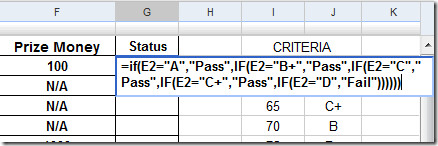The evaluated status of the students is shown in the screenshot below.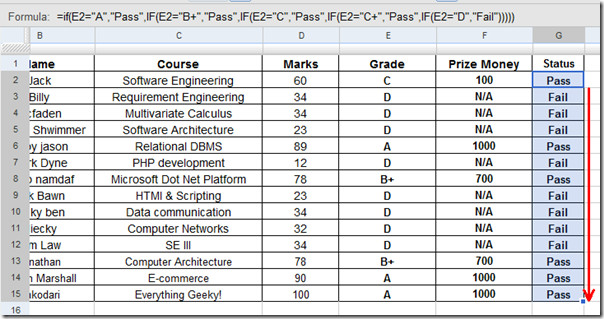With enhanced capabilities of Google Docs, you can now create, collaborate and importantly formulate spreadsheets on the fly without having Excel installed on your system.

1.Benjamin says:

How would you setup a numerical pass or fail? Example: if grade is >= 75, “Pass”, “Fail”
when I try this it just gives error. I am missing something?

•Makroshec says:

Using the example above: =IF(D2 >= 75, “Pass”, “Fail”).
Just be sure that D2 is a value (number) and not a string (text). Even though it “looks” like a number, the spreadsheet evaluates the data by its type, not appearance. I discovered that the newest version of Google Sheets attempts to evaluate both to reduce these errors, but I would not rely on it. There are ways of converting strings to values e.g. VALUE(D2).

2.Diannefair says:

When you add lines to your column the formula doesn’t extend to the new items   Why not, it used to in the earlier version.
I used to just drag the highlighted line down.  That doesn’t work in google docs. I have to keep writng a new formula. HELP

3.EWH says:

=IF(E2=”A”,”Pass”,IF(E2=”B”,”Pass”,IF(E2=”B+”,”Pass”,IF(E2=”C”,”Pass”,IF(E2=”C+”,”Pass”,IF(E2=”D”,”Fail”))))))

equals

=IF(E2=”D”,”Fail”,”Pass”)

Much easier.

•Usman says:

Certainly, using conditional expression is much easier, actually we are just showing that lengthy formulas and famous Excel functions, like VLOOKUP can also be evaluated in Google Docs.

This site uses Akismet to reduce spam. Learn how your comment data is processed.